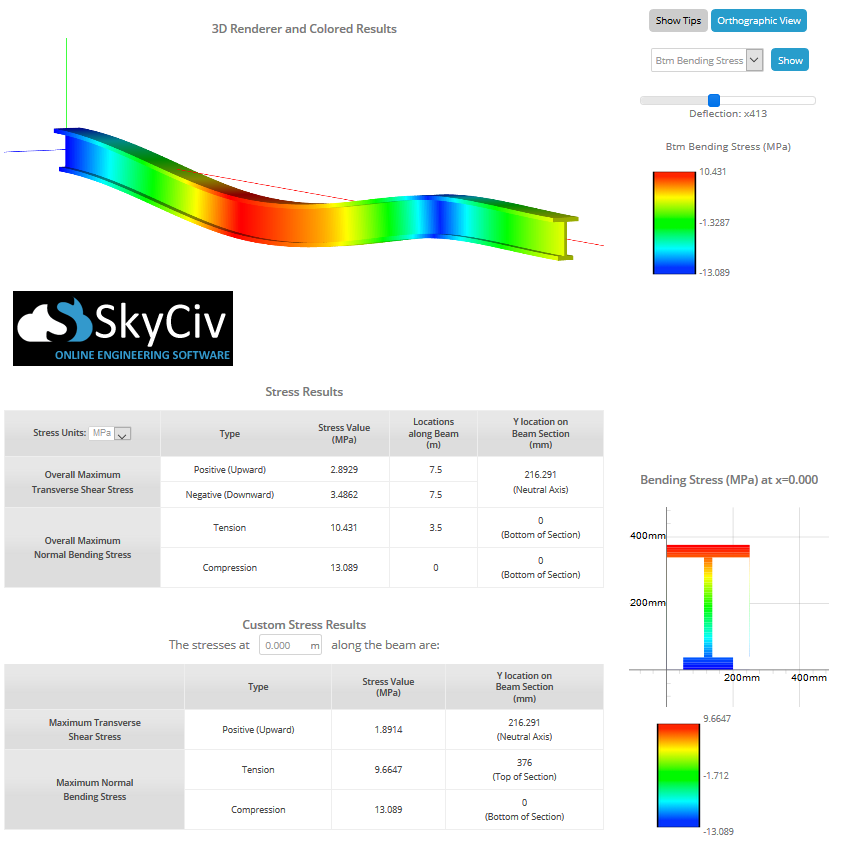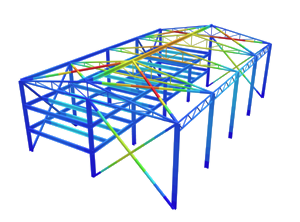SkyCiv Documentation

Your guide to SkyCiv software - tutorials, how-to guides and technical articles

1. Home
2. Tutorials
3. Stress Tutorials
4. Calculate Bending Stress of a Beam Section

# Calculate Bending Stress of a Beam Section

## How to Calculate Bending Stress in Beams

In this tutorial we will look at how to calculate the bending stress of a beam using a bending stress formula that relates the longitudinal stress distribution in a beam to the internal bending moment acting on the beam’s cross section. We assume that the beam’s material is linear-elastic (i.e. Hooke’s Law is applicable). Bending stress is important and since beam bending is often the governing result in beam design, it’s important to understand.

## 1. Calculating by Hand

Let’s look at an example. Consider the I-beam shown below: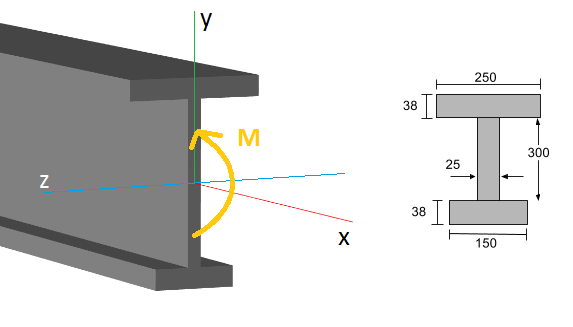At some distance along the beam’s length (the x-axis) it is experiencing an internal bending moment (M) which you would normally find using a bending moment diagram. The general formula for bending or normal stress on the section is given by:

[math]
\sigma_{bend} = \dfrac{My}{I} \text{ where:} \\\\
\begin{align}
M &= \text{the internal bending moment about the section’s neutral axis} \\
y &= \text{the perpendicular distance from the neutral axis to a point on the section} \\
I &= \text{the moment of inertia of the section area about the neutral axis}
\end{align}
[math]

Given a particular beam section, it is obvious to see that the bending stress will be maximised by the distance from the neutral axis (y). Thus, the maximum bending stress will occur either at the TOP or the BOTTOM of the beam section depending on which distance is larger:

[math]
\sigma_{bend,max} = \dfrac{Mc}{I} \text{ where:} \\\\
c = \text{the perpendicular distance from the neutral axis to the farthest point on the section}
[math]

Lets’s consider the real example of our I-beam shown above. In our previous moment of inertia tutorial we already found the moment of inertia about the neutral axis to be I = 4.74×108 mm4. Additionally, in the centroid tutorial we found the centroid and hence the location of the neutral axis to be 216.29 mm from the bottom of the section. This is shown below: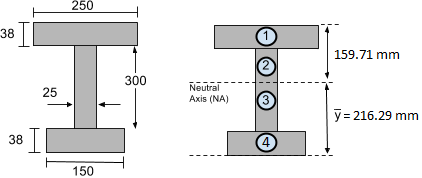Obviously, it is very common to require the MAXIMUM bending stress that the section experiences. For example, say we know from our bending moment diagram that the beam experiences a maximum bending moment of 50 kN-m or 50,000 Nm (converting bending moment units). Then we need to find whether the top or the bottom of the section is furthest from the neutral axis. Clearly the bottom of the section is further away with a distance c = 216.29 mm. We now have enough information to find the maximum stress using the bending stress formula above:

[math]
\sigma_{bend,max} = \dfrac{Mc}{I} \text{ where:} \\\\
\begin{align}
M &= 50 \text{ kNm} = 50,000 \text{ Nm} \\
c &= 216.29\text{ mm} = 0.21629\text{ m} \text{ (BOTTOM)} \\
I &= 4.74\times10^{8} \text{ mm}^{4} = 4.74\times10^{-4} \text{ m}^{4} \\\\
\end{align}
[math]

[math]
\begin{align}
\therefore \sigma_{bend,max} &= \dfrac{(50,000 \text{ Nm})(0.21629\text{ m})}{4.74\times10^{-4} \text{ m}^{4}} \\\\
\sigma_{bend,max} &= 22,815,400 \text{ N/m}^{2} \text{ or Pa} \\
\sigma_{bend,max} &= 22.815 \text { MPa} \\
\end{align}
[math]

Similarly we could find the bending stress at the top of the section, as we know that it is y = 159.71 mm from the neutral axis (NA):

[math]
\begin{align}
\sigma_{bend,top} &= \dfrac{My}{I}\\\\
\sigma_{bend,top} &= \dfrac{(50,000 \text{ Nm})(0.15971\text{ m})}{4.74\times10^{-4} \text{ m}^{4}} \\\\
\sigma_{bend,top} &= 16,847,046 \text{ N/m}^{2} \text{ or Pa} \\
\sigma_{bend,top} &= 16.847 \text { MPa} \\
\end{align}
[math]

The last thing to worry about is whether the stress is causing compression or tension of the section’s fibers. If the beam is sagging like a “U” then the top fibers are in compression (negative stress) while the bottom fibers are in tension (positive stress). If the beam is sagging like an upside-down “U” then it is the other way around: the bottom fibers are in compression and the top fibers are in tension.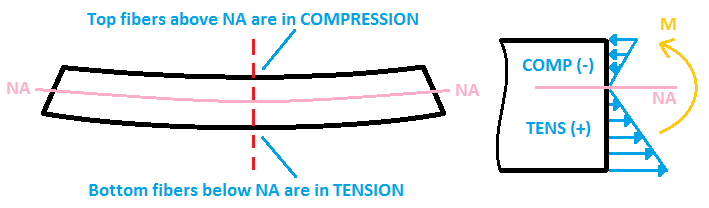## 2. How to Calculate Stress using SkyCiv Beam

Of course you don’t need to do these calculations by hand because you can use the SkyCiv Beam – bending stress calculator to find shear and bending stress in a beam! Simply start by modeling the beam, with supports and apply loads. Once you hit solve, the sftware will show the max stresses from this bending stress calculator. The image below shows an example of an I-beam experiencing bending stress: